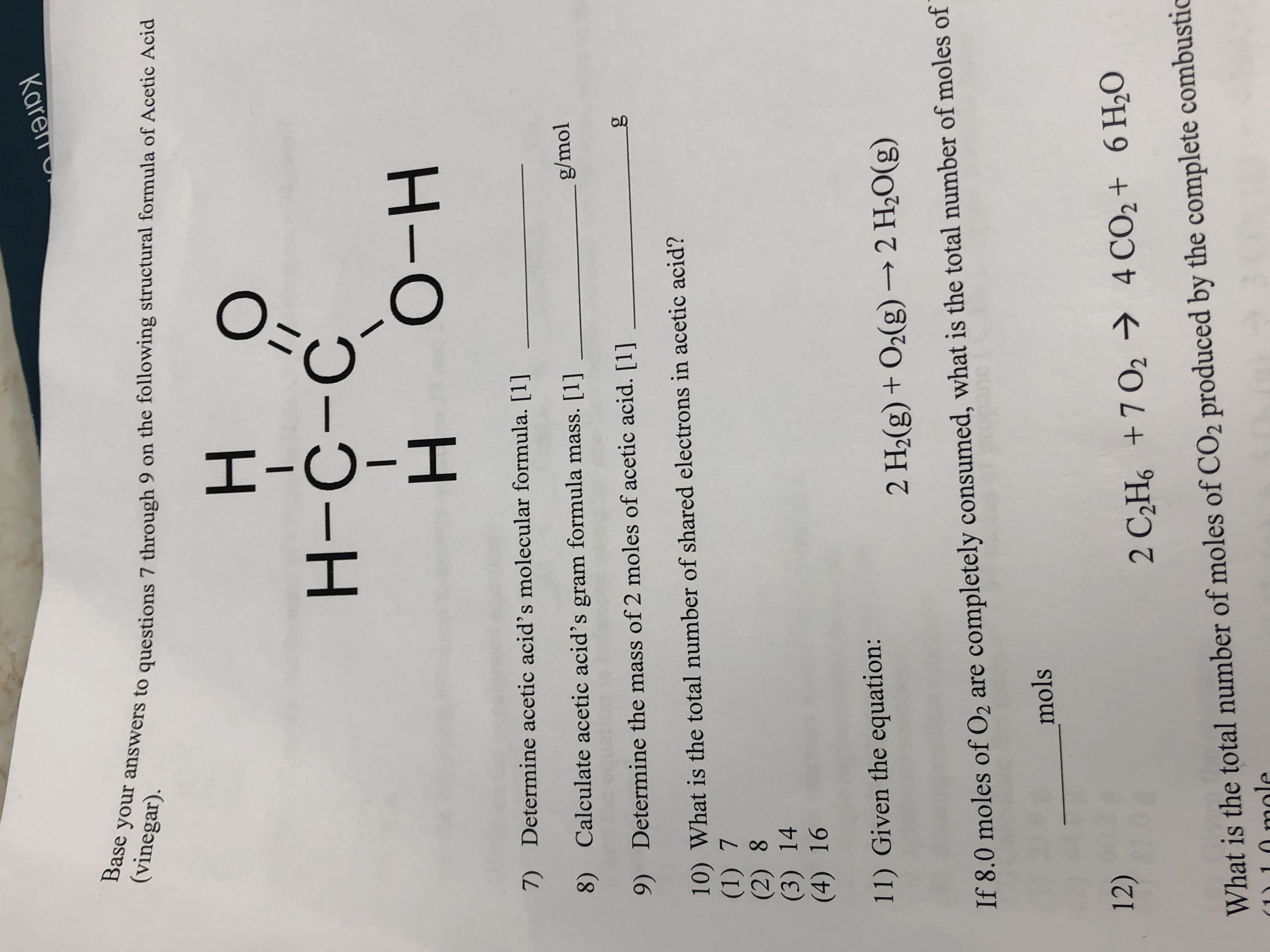# KarenBase your answers to questions 7 through 9 on the following structural formula of Acetic Acid(vinegar).н-о-нDetermine acetic acid's molecular formula. 7)g/molCalculate acetic acid's gram formula mass. 8)gDetermine the mass of 2 moles of acetic acid. 9)10) What is the total number of shared electrons in acetic acid?(1) 7(2) 8(3) 14(4) 1611) Given the equation:2 H2(g) + O2(g)2 H20(g)If 8.0 moles of O2 are completely consumed, what is the total number of moles ofmols12)4 CO2+ 6 H202 C2Hs+7 02What is the total number of moles of CO2 produced by the complete combusticn moleO=I-O-I

Question
1 views

Question 7help_outlineImage TranscriptioncloseKaren Base your answers to questions 7 through 9 on the following structural formula of Acetic Acid (vinegar). н- о-н Determine acetic acid's molecular formula.  7) g/mol Calculate acetic acid's gram formula mass.  8) g Determine the mass of 2 moles of acetic acid.  9) 10) What is the total number of shared electrons in acetic acid? (1) 7 (2) 8 (3) 14 (4) 16 11) Given the equation: 2 H2(g) + O2(g)2 H20(g) If 8.0 moles of O2 are completely consumed, what is the total number of moles of mols 12) 4 CO2+ 6 H20 2 C2Hs+7 02 What is the total number of moles of CO2 produced by the complete combustic n mole O= I-O-I fullscreen
check_circle

Step 1

The molecular formula of acetic acid has to be determined.

Molecular formula:

The molecular formula is the chemical representation of a compound in which the comp...

### Want to see the full answer?

See Solution

#### Want to see this answer and more?

Solutions are written by subject experts who are available 24/7. Questions are typically answered within 1 hour.*

See Solution
*Response times may vary by subject and question.
Tagged in

### General Chemistry